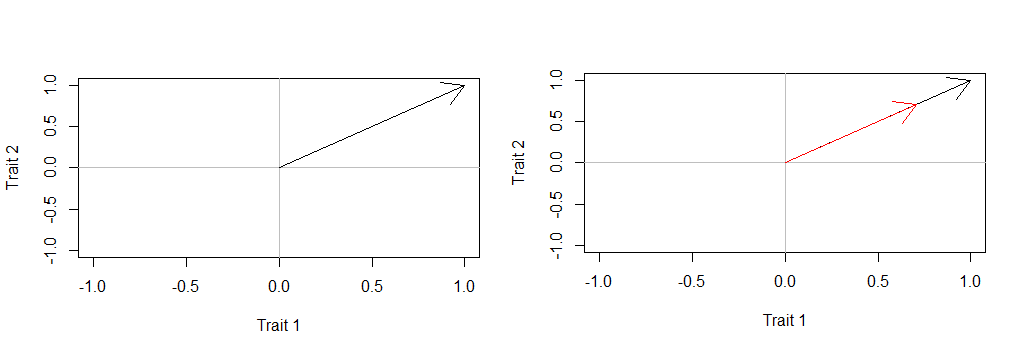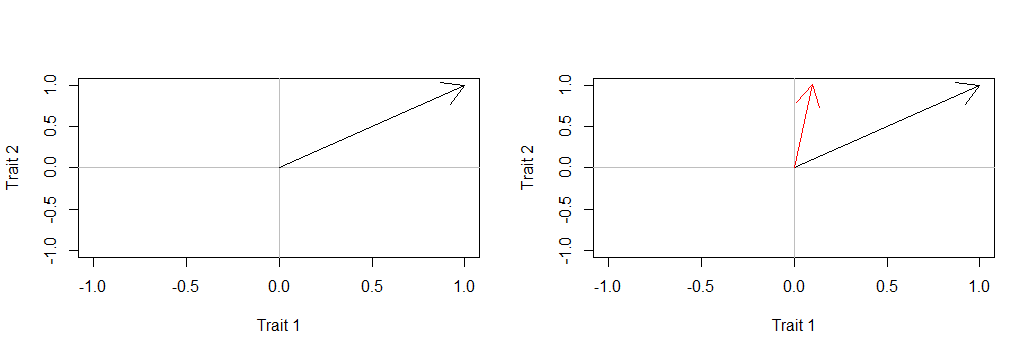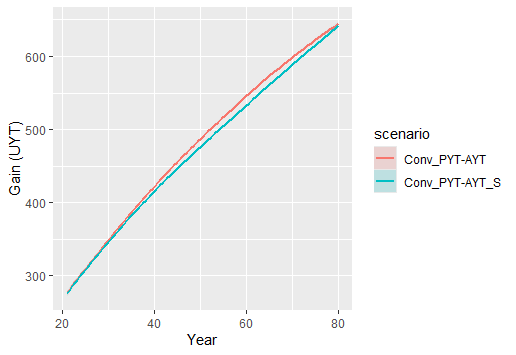January 26th, 2021

## Assumptions

1. Phenotypes and breeding values are multivariate normal

 𝑍~𝑁(µ, Σ)
2. Weights (b): explicitly or implicitly assigned

traitNames <- c(“MCMDS”, “DM”, “HI”, “RTSZ”, “PLTHT”, “FYLD”)

econWt (b) = c(-10, 20, 7, 10, 10, 20)

3. A univariate linear index

$$𝐼=Σ𝑏_𝑖 𝑧_𝑖=𝑏^𝑇 z$$

4. A constant economic model

## 1.0 Scaling Weights

An index weight is a vector with direction and length:

e.g. econWt (b) = c(-10, 20, 7, 10, 10, 20)

Only the direction is important in the selection scheme and not the length

Therefore, the length of weights can be rescaled without affecting the desired selection, e.g to scale the weight of FYLD to 1, we can divide all weights by an appropriate amount.

## 1.1 Example

Simulate two traits

Index weights(w)= c(1,1)

Smith-Hazel index

$$𝐼_𝑠=𝑏_𝑠^𝑇 z$$ , where $$𝑏_𝑠 =𝑃^−1 𝐺𝑎$$

Base index (independent from P and G matrices)

$$𝐼_𝑏= 𝑎^𝑇 𝑧$$

### Treatments

Treatment Description
Unscaled_Weights w = c(1,1), use either Smith-Hazel or Base index
Scaled_Weights The current weight (w) scaled to one (w_s = w/sqrt(sum(w^2))), either Smith-Hazel or Base index

## 1.2 Scaling an index does not change desired selection

Comparing selection direction of unscaled vs scaled index weightsScaling the length of an index does not affect desired selection. The ranking of the genotypes and the desired selection direction remains the same despite the shortened index length

## 2.0 Scaling traits

There is a connection between scaling weights and scaling traits

Scaling one is equivalent to scaling the other.

Therefore, it is possible to change the direction of selection through scaling of traits

## 2.1 Example

Two random traits with different variances

Scale the traits

Keep initial weights

Correlate the base index for scaled and unscaled traits

Show the equivalency of scaling the index and scaling the trait

### Treatments

Treatment Description
Unscaled_traits Y = Y, w = c(1,1), Base index
Scaled_traits Y = Y_s, w = c(1,1), Base index

## 2.2 Scaling traits can change the desired selection

Comparing unscaled and scaled traits using the same index weightsScaling traits can change the direction of selection. Therefore, scaling a trait is equivalent to scaling an index but in this case the index can change direction.

## 3.0 Simulation based on Next-Gen Pipeline

### Treatments

Treatment Description
Conv_PYT-AYT ADG traits, no GS, recycling using the proposed mixed crossing block from both PYT and AYT, scale = FALSE
Conv_PYT-AYT_S ADG traits, no GS, recycling using the proposed mixed crossing block from both PYT and AYT, scale = TRUE

#### Simulation procedure

A 20 year burn-in period was modeled using the baseline. The burn-in was followed by a 60 year evaluation period. Genetic gain was measured by assessing changes in genetic merit at UYT. Genotype-by-year interaction variance was assumed to be equivalent to genetic variance (based on average correlation between locations being equal to 0.5). 10 replications done. traitsNames = c(“MCMDS”, “DM”, “HI”, “RTSZ”, “PLTHT”, “FYLD”) and econWt = c(-10, 20, 7, 10, 10, 20).

## 3.1 Results

Comparing genetic merit for the index based on scaled and unscaled traits## 4. Conclusion

There is a risk of constantly changing the direction of selection by scaling traits because scaling is data dependent. We do not recommend

When selecting based on phenotypes, it is better to use Smith-Hazel weights that can be adjusted when variances change, while keeping the economic weights constant

Better still, is to apply economic weights directly to BLUPs without calculating the Smith-Hazel weights because BLUP estimation ensures that changes in variance are captured in the variance changes of BLUPs

However, if the program is already using scaled traits with the current weights, it is not advisable to just stop and assume un-scaled traits and vice-versa. There will be need to readjust the weights in order to achieve the desired selection direction

Among other assumptions, it is assumed that the economic model is constant and the current weights are correct. However, occasional restrospective index calculation using effective selection differentials may help determine how natural selection is affecting the desired selection scheme